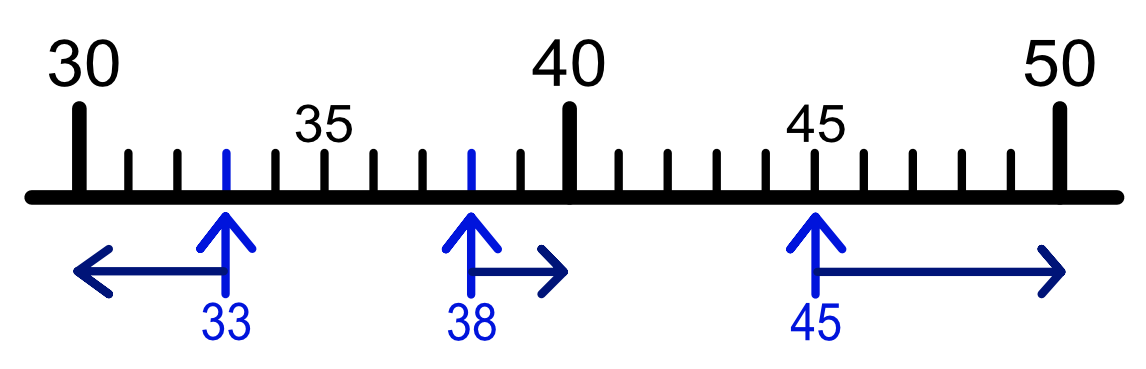Rounding to the Nearest 10, 100, 100

## Rounding to the Nearest 10, 100, 100

Rounding gives an approximate answer. For example, London to Manchester is 208 miles; but it is easier to remember it as 200 miles.

When rounding to the nearest 10, check if the last digit is 5 or more. If it is, move the value up to the next multiple of 10. If the last digit is less than 5, move the value of the whole number down to the previous multiple of 10.

For the number 38, the last digit is an 8, which is greater than 5, so 38 becomes 40. The last digit in 33 is a 3, so it rounds down to 30.

The last digit of 45 is a 5. The rule is that if it is 5 or more it rounds up. To the nearest 10, 45 rounds to 50.If a number is over 100, it can still be rounded to a nearest ten. The last digit of 3467 is a 7, so it rounds up to the nearest 10s value which is 3470.

Numbers can be rounded to the nearest 100. This time, the half-way point is the value 50. If the last two digits are less than 50, th number will round down. If the last two digits are 50 or greater, the number rounds up. The number 456 rounds up to 500 as the "56" part is greater than 50; and 432 (the "32" rounds the number down to 400).

Numbers can be rounded to the nearest 1000: the half-way point is 500. Examining 8462 shows that the "462" part is less than 500, so 8462 rounds down to 8000.

Some examples of rounding numbers are given below:

 number 852 2 465 48 566 to nearest 10 850 2 470 48 570 to nearest 100 900 2 500 48 600 to nearest 1000 1 000 2 000 49 000

## Example 1

A concert hall has seats for 4425 people. How many is that to the nearest 100?

The number is between 4400 and 4500; 4425 is below the half-way point (4450) between these two numbers, so it rounds down.